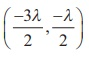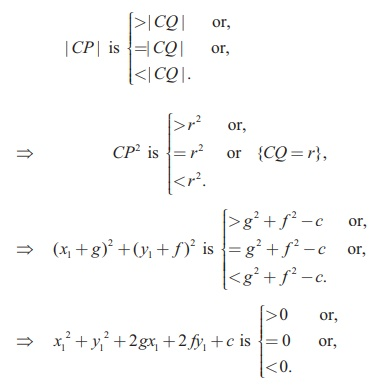Home | | Maths 12th Std | Circle: Solved Example Problems

# Circle: Solved Example Problems

Equation of a circle in standard form, Condition for the line y = mx + c to be a tangent to the circle and finding the point of contact

## Equation of a circle in standard form

Example 5.1

Find the general equation of a circle with centre (−3, −4) and radius 3 units.

Solution

Equation of the circle in standard form is  ( x - h)2  + ( y - k )2  = r 2

(x - (-3))2  + ( y - (-4))2  = 32

( x + 3)2  + ( y + 4)2  = 32

x2 + y2 + 6+ 8 +16 = 0 .

Example 5.2

Find the equation of the circle described on the chord 3+ 5 = 0 of the circle x2 + y2 = 16 as diameter.

Solution

Equation of the circle passing through the points of intersection of the chord and circle by Theorem 5.1 is x2 + y2 -16 + l (3+ 5) = 0 .

The chord 3+ 5 = 0 is a diameter of this circle if the centrelies on the chord.Therefore, the equation of the required circle is x2 + y2 + 3-11 = 0.

Example 5.3

Determine whether −1 = 0 is the equation of a diameter of the circle x2 + y2 − 6+ 4 = 0 for all possible values of .

Solution

Centre of the circle is (3, -2) which lies on -1 = 0 . So the line -1 = 0 passes through the centre and therefore the line -1 = 0 is a diameter of the circle for all possible values of .

Theorem 5.2

The equation of a circle with (x1 , y1 ) and (x2 , y2 ) as extremities of one of the diameters of the circle is (− x1 )(− x2 ) + ( − y1)( − y2) = 0.

Proof

Let A(x1 , y1 ) and B(x2 , y2 ) be the two extremities of the diameter AB , and P(xy) be any point on the circle. Then ÐAPB = π/2 (angle in a semi-circle).Therefore, the product of slopes of AP and PB is equal to -1.

That is,= −1 yielding the equation of the required circle as

(x1 )(x2 ) + ( y1 )( y2 ) = 0 .

Example 5.4

Find the general equation of the circle whose diameter is the line segment joining the points (−4, −2) and (1,1) .

Solution

Equation of the circle with end points of the diameter as( x1 , y1 ) and ( x2 , y2 ) given in

theorem 5.2 is

x1 )( x2 ) + ( y1 )( y2 ) = 0

( + 4)( -1) + ( + 2)( -1) = 0

⇒ x2 + y2 + 3- 6 = 0 which is the required equation of the circle.

Theorem 5.3

The position of a point P(x1 , y1 ) with respect to a given circle x2 + y2 + 2gx + 2 fy + = 0 in the Plane containing the circle is Outside or on or inside the circle according as x2 + y2 + 2gx + 2 fy + isProof

Equation of the circle is x2 + y2 + 2gx + 2 fy = 0 with centre C (-g, - ) and radiusLet P(x1y1 ) be a point in the plane. Join CP and let it meet the circle at .Then the point is outside, on or within the circle according asExample 5.5

Examine the position of the point (2, 3) with respect to the circle x2 + y2 − 6− 8 +12 = 0.

Solution

Taking (x1y1) as (2, 3), we get

x2 + y2 + 2gx1 + 2 fy1 = 22 + 32 - 6 ´ 2 - 8´ 3 +12 ,

= 4 + 9 -12 - 24 + 12 = -11 < 0.

Therefore, the point (2, 3) lies inside the circle, by theorem 5.3.

Example 5.6

The line 3+ 4 −12 = 0 meets the coordinate axes at and . Find the equation of the circle drawn on AB as diameter.

Solution

Writing the line 3+ 4= 12, in intercept form yields x/4 y/3 = 1. Hence the points and are (4, 0) and(0, 3) .

Equation of the circle in diameter form is

x1 )( x2 ) + ( y1 )( y2 ) = 0

- 4)( - 0) + ( - 0)( - 3) = 0

x2 + y2 - 4- 3y = 0

Example 5.7

A line 3+ 4 +10 = 0 cuts a chord of length 6 units on a circle with centre of the circle (2,1) . Find the equation of the circle in general form.

Solution

C(2,1) is the centre and 3+ 4 +10 = 0 cuts a chord AB on the circle. Let be the midpoint of AB . Then we have

AM  = BM = 3 . Now  BMC is a right triangle.

So, we have CM  == 4.

By Pythogoras theorem BC2 = BM2 + MC2  = 32 + 42 = 25So, the equation of the required circle is (- 2)2 + ( -1) = 52

x2 + y2 - 4- 2 – 20 = 0.

Example 5.8

A circle of radius 3 units touches both the axes. Find the equations of all possible circles formed in the general form.

Solution

As the circle touches both the axes, the distance of the centre from  both the axes is 3 units, centre can be (±3, ±3) and hence there are four circles with radius 3, and the required equations of the four circles are x2 + y2 ± 6± 6 + 9 = 0 .Example 5.9

Find the centre and radius of the circle 3x2 + (+1) y2 + 6- 9+ 4 = 0.

Solution

Coefficient of x2 = Coefficient of y2 (characteristic (ii) for a second degree equation to represent a circle).

That is, 3 = +1 and = 2 .

Therefore, the equation of the circle is

3x2 + 3y2 + 6- 9 + 6 = 0

x2 + y2 + 2- 3+ 2 = 0

So, centre is ( -1, 3/2 ) and radius r =Example 5.10

Find the equation of the circle passing through the points (1,1), (2, −1) , and (3, 2) .

Solution

Let the general equation of the circle be

x2 + y2 + 2gx + 2 fy = 0        ……….(1)

It passes through points (1,1), (2, -1) and (3, 2) .

Therefore, 2+ 2 c = -2 , … (2)Therefore, the required equation of the circle isÞ x2 + y2 - 5+ 4 = 0

Note

Three points on a circle determine the equation to the circle uniquely. Conversely three equidistant points from a centre point forms a circle.

## Condition for the line y = mx + c to be a tangent to the circle x2+ y2 = a2 and finding the point of contact

Example 5.11

Find the equations of the tangent and normal to the circle x2 + y2 = 25 at P(−3, 4) .

Solution

Equation of tangent to the circle at P(x1 , y1 ) is xx1 + yy1 = a2

That is, x(-3) + y(4) = 25

-3+ 4 = 25

Equation of normal is xy1 - yx1 = 0

That is, 4+ 3= 0 .

Example 5.12

If = 4is a tangent to the circle x2 + y2 = 9 , find c.

Solution

The condition for the line mx to be a tangent to the circle x2 + y2 = a2 is c2 = a2 (1+ m2).

Then, c = ± √[9(1+16)]

c = ±3 √17.

Example 5.13

A road bridge over an irrigation canal have two semi circular vents each with a span of 20and the supporting pillars of width 2. Use Fig.5.16 to write the equations that represent the semi-verticular vents.Solution

Let  O1 O2  be  the  centres  of  the two semi circular vents.

First vent with centre O1 (12, 0) and radius = 10 yields equation to first semicircle as

(-12)2 + ( - 0)2 = 102

Þ x2 + y2 - 24+ 44 = 0 , > 0 .

Second vent with centre O2 (34, 0) and radius = 10 yields equation to second vent as

(- 34)2 + y2 = 102

Þ x2 + y2 - 68+1056 = 0 , > 0 .

Tags : with Answers, Solution , 12th Mathematics : UNIT 5 : Two Dimensional Analytical Geometry II
Study Material, Lecturing Notes, Assignment, Reference, Wiki description explanation, brief detail
12th Mathematics : UNIT 5 : Two Dimensional Analytical Geometry II : Circle: Solved Example Problems | with Answers, Solution